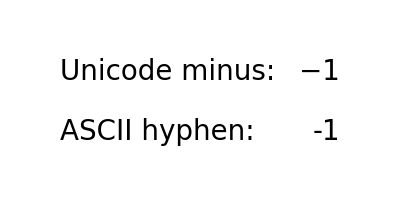# Unicode minus#

By default, tick labels at negative values are rendered using a Unicode minus (U+2212) rather than an ASCII hyphen (U+002D). This can be controlled by setting rcParams["axes.unicode_minus"] (default: True).

The replacement is performed at draw time of the tick labels (usually during a pyplot.show() or pyplot.savefig() call). Therefore, all tick labels of the figure follow the same setting and we cannot demonstrate both glyphs on real tick labels of the same figure simultaneously.

Instead, this example simply showcases the difference between the two glyphs in a magnified font.import matplotlib.pyplot as plt

fig = plt.figure(figsize=(4, 2))
fig.text(.15, .6, "Unicode minus:", fontsize=20)
fig.text(.85, .6, "\N{MINUS SIGN}1", ha='right', fontsize=20)
fig.text(.15, .3, "ASCII hyphen:", fontsize=20)
fig.text(.85, .3, "-1", ha='right', fontsize=20)
plt.show()


Gallery generated by Sphinx-Gallery